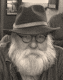# FOLIO OLIOI'm an artist, educator, militant anti-theist , and I write. I gamble on just about anything. And I like beer...but I love my wife. This blog contains observations from a funny old man who gets pissed off every once in a while.

## Saturday, August 15, 2020

David said...

117Anonymous said...

Solution = 810Anonymous said...

414Anonymous said...

Puzzle time....
This is intriguing because in the equation defining a clock, the third clock is the mirror image or possibly ? inverse of the other 2. Thus, the "inverse" clock could be 1/clock. Or perhaps it is negative of clock. Or perhaps the first two clocks, where the time is equal to 9, their value is 9 and the third clock value is 3.
Presuming 1/clock is represented by the 3rd clock, this means that clock + clock + 1/clock = 21. Using the quadratic equation, the value of clock would be 10.45 or roughly 10.5. Or it could be roughly 0.05.

If the third clock is interpreted as negative clock, then clock is simply 21.

If the values of the clocks are represented as time, then the first two clocks are each 9, and the third clock is 3.(9+9+3 = 21). This is the simplest interpretation.

Now we have the calculator. 3 of the "1234" calculators equals 30. Thus, each "1234" calculator equals 10. The sum of 1+2+3+4 = 10. One can assume the value of the calculator is simply the sum of the numbers displayed in the window.

Then we have the light bulbs. 2 light bulbs minus a light bulb equals 15. That means a light bulb is equal to 15. But those are all light bulbs with 5 hash marks. One could assume then that each hash mark refers to a multiple of 3, such that 5 hash marks times 3 = 15. Or, the light bulb with no mark is 10, and each hash mark means you add 1. I like this version better because it is simpler...only involves adding vs. multiplication.

Having said all this, using the simplest interpretations, we have the following for the equation to solve:
Clock = 9 (time on clock is 9)
calculator value = 9 (1+2+2+4)
each light bulb = bulb + 4 hashes = 10 + 4 = 14
we have 3 light bulbs...assume we would add all 3 together = 42 (14+14+14)
using correct order of operations, multiply by calculator value = 9*42 = 378
finally, add a clock = 378 + 9 = 387

Answer is 387, using the simplest interpretations of the symbols. But I would argue there are multiple valid answers.

Stephen Hait said...

Puzzle: 457
Clock=7
Calc=10
Bulb=15
Clock + (Calc * 3(15))=
7 + (10 * 45)-
7 + 450)=457Anonymous said...

Puzzle:. My answer is 459. My reasoning is as follows. If it is inaccurate, I would appreciate enlightenment.
1. The clocks are not the same. If you use the position of the hands as
numbers, you find that 9 + 9 + 3 = 21
2. The calculators are the same, so 10+10+10 = 30, and one calculator would
equal 10.
3. The second two bulbs cancel each other, so one bulb = 15. 15+15-15=15
4. The order of operations in an equation can be remembered by the acronym
"PEMDAS", meaning that the components of an equation should be solved
in the following order: parenthesis, exponents, multiplication,
excuse my dear aunt Sally".
6. Therefore,
A. Three light bulbs at 15 each would equal 45
B. 10 X 45 = 450
C. 9 + 450 = 459
D. The equation would be 9 + (10 X (15 X 3))

7. Of course, if you view the three light bulbs as 15 X 15 X 15 instead of
15 X 3, you get a different answer. The equation then becomes:
9 + (10 X (15 X 15 X 15)), although I doubt this is implied by the three
bulbs.

Ralph Henry said...

NOW THAT IS HOW TO SOLVE A PROBLEM!!!

Stephen Hait said...

Puzzle: Third try
Two different clocks - 9 o'clock (2) and 3 o'clock (1)= 21
So 9 + 9 + 3 = 21
So clocks value based on time
Calculators - all read 1234 total 30
So 1+2+3+4 = 10 * 3 clocks = 30
So Calculator based on sum of numbers in display
Bulbs each have 5 ticks so 5 ticks * 3 = 15
So bulb value = 3 x number of ticks (5 each)
Clock (9 o'clock = 9) + (Calc [1224 - total of digits is 9] * 3 bulbs each with 4 ticks at 3 per tick = 12)
So 9 (clock) + (9 [calc sum of display numbers] * 36 [bulb - 4 ticks at 3/tick * 3 =36])
Or 9 + (9 * 36)
9 + 324 = 333Anonymous said...

To the person who answered 387...that was a brilliant analysis. I wrote the 457 comment, and learned to consider further details from your answer instead of thinking things remained the same. That is a good life lesson. Thanks.Anonymous said...

I did it the same way as the one who answered 459 above, but my answer is 414 because the calculator has a value of 9 not 10.Danny Newey said...

A6- That cloud probably weighs thousands of Tons! I'd say AT LEAST 5 thousand for a storm that size!

## Random Post

Random Posts Widget